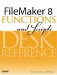# Random

Category: Number

 Syntax: Random

Parameters: None

Data type returned: Number

Description:

Returns a random decimal between zero and one (but will not return 1 nor 0.) When used in a field definition, a new value is generated when the formula is updated. It is also updated anytime the formula re-evaluates, such as when other field values referenced in the formula change. In an unstored calculation, the Random() function re-evaluates each time the field is displayed.

If you want to generate a random integer in a certain range, multiply the result returned by the random function by the range you want to produce; then use the Int(), Floor(), Ceiling(), Round(), or truncate() functions to remove the decimals.

For instance, to return a random number from 1 to 6 (as in the roll of a die), use the formula:

``` Ceiling (Random * 6)
```

If you need to specify a lower bound for the random number, just add the bound to the results of the random number. For instance, to return a random number between 10 and 100, inclusive, use the following formula:

```Int (Random * 91) + 10
```

Examples:

Function

Results

Round (Random; 5)

Might return .07156.FileMaker 8 Functions and Scripts Desk Reference
ISBN: 0789735113
EAN: 2147483647
Year: 2004
Pages: 352